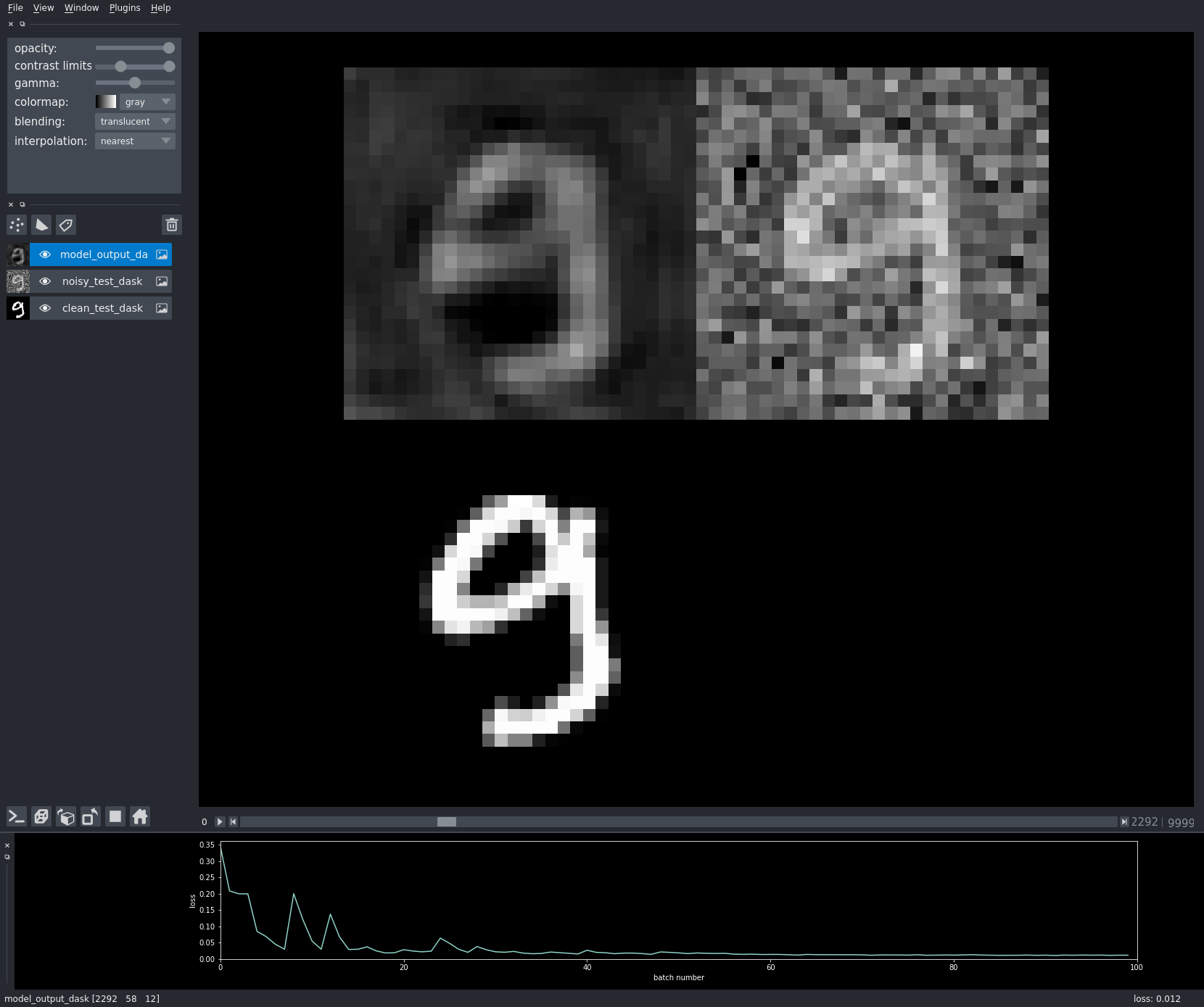# Noise2Self for Neural Nets¶

This is a simple notebook demonstrating the principle of using self-supervision to train denoising networks.

For didactic purposes, we use a simple dataset (Gaussian noise on MNIST), a simple model (a small UNet), and a short training (100 iterations on a CPU). This notebook runs on a MacBook Pro in under one minute.

In :
%gui qt

In :
import sys
sys.path.append("..")

In :
from util import show, plot_images, plot_tensors


# Data¶

We demonstrate the use of a self-supervised denoising objective on a synthetically noised version of MNIST.

In :
import numpy as np

from torchvision.datasets import MNIST
from torchvision import transforms
from torch.utils.data import Dataset

transform = transforms.Compose([
transforms.ToTensor(),
]), train = True)

transform = transforms.Compose([
transforms.ToTensor(),
]), train = False)

In :
from torch import randn
return img + randn(img.size())*0.4

class SyntheticNoiseDataset(Dataset):
def __init__(self, data, mode='train'):
self.mode = mode
self.data = data

def __len__(self):
return len(self.data)

def __getitem__(self, index):
img = self.data[index]

In :
noisy_mnist_train = SyntheticNoiseDataset(mnist_train, 'train')
noisy_mnist_test = SyntheticNoiseDataset(mnist_test, 'test')


We will try to learn to predict the clean image on the right from the noisy image on the left.

In :
noisy, clean = noisy_mnist_train
plot_tensors([noisy, clean], ['Noisy Image', 'Clean Image'])


The strategy is to train a $J$-invariant version of a neural net by replacing a grid of pixels with the average of their neighbors, then only evaluating the model on the masked pixels.

In :
from mask import Masker

In :
net_input, mask = masker.mask(noisy.unsqueeze(0), 0)


A mask; the data; the input to the neural net, which doesn't depend on the values of $x$ inside the mask; and the difference between the neural net input and $x$.

In :
plot_tensors([mask, noisy, net_input, net_input - noisy],
["Mask", "Noisy Image", "Neural Net Input", "Difference"])


# Model¶

For our model, we use a short UNet with two levels of up- and down- sampling

In :
from models.babyunet import BabyUnet
model = BabyUnet()


# Training¶

In :
from torch.nn import MSELoss

loss_function = MSELoss()


# Visualization¶

We lazily convert the torch tensors to NumPy arrays and concatenate them into dask arrays containing all the data. We do this for the training (noisy) data, the ground truth, and the model output.

There's a bit of reshaping because torch data comes with extra dimensions that we want to squeeze out, to only get a (nsamples, size_y, size_x) volume.

In :
import dask

[
da.from_delayed(
shape=(1, 28, 28),
dtype=np.float32
).reshape((28, 28))
for i in range(len(noisy_mnist_test))
]
)

[
da.from_delayed(
shape=(1, 28, 28),
dtype=np.float32
).reshape((28, 28))
for i in range(len(noisy_mnist_test))
]
)

In :
import torch

def test_numpy_to_result_numpy(i):
"""Convert test NumPy array to model output and back to NumPy."""
out = model(
).detach().numpy().squeeze()
return out

# build the results dask array
[
da.from_delayed(
shape=(28, 28),
dtype=np.float32
)
for i in range(len(noisy_mnist_test))
]
)


Build the napari viewer for all three volumes simultaneously:

In :
import napari

viewer = napari.Viewer()
contrast_limits=(
)
)  # this layer though, we're gonna play with
viewer.grid_view()


We turn off dask caching because we want the model to re-evaluate each time we view a model output.

In :
from napari.utils import resize_dask_cache

Out:
<dask.cache.Cache at 0x7ff4ea8332b0>

Finally, build a loss plot and refresh the viewer on each batch:

In :
from napari.qt import thread_worker
from matplotlib.backends.backend_qt5agg import FigureCanvas
from matplotlib.figure import Figure

NUM_ITER = 100

# build the plot, but don't display it yet
# — we'll add it to the napari viewer later
with plt.style.context('dark_background'):
loss_canvas = FigureCanvas(Figure(figsize=(5, 3)))
loss_axes = loss_canvas.figure.subplots()
lines = loss_axes.plot([], [])  # make empty plot
loss_axes.set_xlim(0, NUM_ITER)
loss_axes.set_xlabel('batch number')
loss_axes.set_ylabel('loss')
loss_canvas.figure.tight_layout()
loss_line = lines

# when getting a new loss, update the plot
def update_plot(loss):
x, y = loss_line.get_data()
new_y = np.append(y, loss)
new_x = np.arange(len(new_y))
loss_line.set_data(new_x, new_y)
loss_axes.set_ylim(
np.min(new_y) * (-0.05), np.max(new_y) * (1.05)
)
loss_canvas.draw()

# and update the model output layer
def update_viewer(loss):
model_layer.refresh()
viewer.help = f'loss: {loss}'

# define a function to train the model in a new thread,
# connecting the yielded loss values to our update functions

for i, batch in zip(range(n_iter), data_loader):
noisy_images, clean_images = batch

net_output = model(net_input)

loss.backward()

optimizer.step()

yield round(loss.item(), 4)

# finally, add the plot to the viewer, and start training!


In :
from napari.utils import nbscreenshot

nbscreenshot(viewer)

Out:# J-invariant reconstruction¶

With our trained model, we have a choice. We may do a full $J$-invariant reconstruction, or we may just run the noisy data through the network unaltered.

In [ ]:
test_data_loader = DataLoader(noisy_mnist_test,
batch_size=32,
shuffle=False,
num_workers=3)
noisy, clean = test_batch

In [ ]:
simple_output = model(noisy)

In [ ]:
type(simple_output)

In [ ]:
np.asarray(simple_output)


torch.Tensor.__array__ is hampered because the developers want to prevent accidentally dropping gradient. See:

https://discuss.pytorch.org/t/should-it-really-be-necessary-to-do-var-detach-cpu-numpy/35489

For demo purposes, we increase the magic of torch Tensors.

In [ ]:
import torch

In [ ]:
def array(self, dtype=None):
if dtype is None:
return self.detach().numpy()
else:
return self.detach().numpy().astype(dtype, copy=False)

torch.Tensor.__array__ = array

In [ ]:
np.array(simple_output)

In [ ]:
import napari

viewer = napari.Viewer()

noisy, name='noisy',
contrast_limits=[-1.5, 2.5],
)